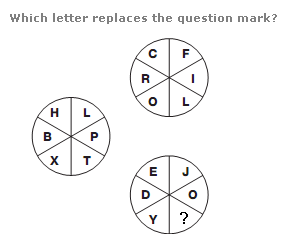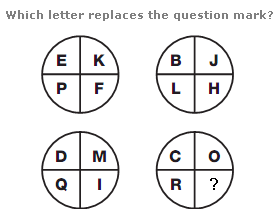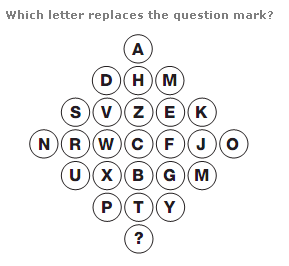# Puzzles - Missing letters puzzles

### Exercise :: Missing letters puzzlesAnswer : T Explanation : Starting with the top left segment of each circle, and moving clockwise, letters follow the alphabetic sequence, in steps of 3 letters for the top circle, 4 letters for the left hand circle, and 5 letters for the bottom circle.Answer : L Explanation : In each circle, the numerical value of the letter in the lower left quarter equals the sum of the numerical values of the upper 2 letters, and the numerical value of the letter in the lower right quarter equals the difference between the numerical values of the upper 2 letters.Answer : E Explanation : Start at the top of the diagram, and work through each row from left to right. Letters move through the alphabet, In steps of 3 letters, then 4, 5 and 6, repeating this sequence